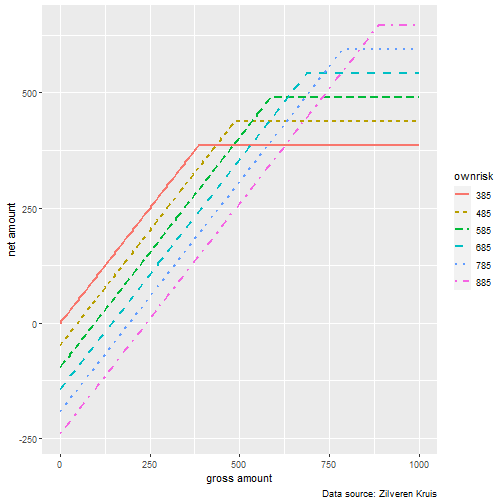# Gross and net excess (eigen risico) in Dutch health care costs

## 2020/10/10

### Load packages that will be used

HOQCutil::silent_library(c('knitr','tidyr','ggplot2'))


### Introduction

The Dutch healthcare system repays insured individuals the costs they have made for health reasons. Of course there are lots of details but the article Understanding ‘Eigen Risico’ gives an introduction.
Only costs above a certain amount (the excess that is called ‘eigen risico’ in Dutch) are repaid. The standard excess is 385 euros but an individual can choose a regime with an higher amount and is then compensated by a reduction of the premium according to Table 1:

er  <- 285+100*(1:6)
pr  <- -48 + 48*(1:6)

regime excess reduction
1 385 0
2 485 48
3 585 96
4 685 144
5 785 192
6 885 240
Table 1: yearly excess amount with premium reduction

### Net amount to pay for health costs

For each gross amount each regime has a corresponding net amount:

net_amount <- function (gross_amount,regime) {
er1 = er[regime]
pr1 = pr[regime]
pmin(gross_amount,er1) - pr1
}


### Calculate net amount for gross amount in the range [0, 1000] for each regime

The function fun calculates the net amounts for one regime and y contains these for all regimes in a list. The results are placed in data.frame df1.

gross_amounts <- seq.int(0,1000,5)
fun <- function (regime) {
purrr::map_dbl(gross_amounts,~net_amount(.,regime))
}
y <- purrr::map(1:6,fun)
df1 <- data.frame(
x=gross_amounts,
er385= y[], er485= y[], er585= y[],
er685= y[], er785= y[], er885= y[]
)


### Create plot after making data.frame ‘long’

The columns with names starting with ‘er’ are then ‘gathered’ in the field ownrisk where these characters are omitted

df1L <-tidyr::pivot_longer(df1,er385:er885,names_to='ownrisk',names_pattern='er(.*)' )
p=ggplot(data=df1L,aes(x,value,group=ownrisk,color=ownrisk,linetype=ownrisk)) +
geom_line(size=1) +
labs( caption = "Data source: Zilveren Kruis",
x="gross amount", y = "net amount") +
theme(
plot.title = element_text(hjust = 0.5),
plot.subtitle = element_text(hjust = 0.5)
)
pFigure 1: Net for gross schedule per ownrisk (excess) regime

### Conclusion

From Figure 1 we see that despite the existence of the various regimes there are only two optimal regimes:

• regime 6 (excess amount 885) when the gross amount is less or equal than 625. For gross amount = 625 the net amount is 625 - 240 = 385
• regime 1 (excess amount 385) when the gross amount is greater or equal than 625. For gross amount = 625 the net amount is 385 - 0 = 385

So the problem of determining an optimal excess amount (eigen risico) is reduced to estimating beforehand if the gross amount is more or less than 625 euros in a year.

## Session Info

This document was produced on 10Oct2020 with the following R environment:

  #> R version 4.0.2 (2020-06-22)
#> Platform: x86_64-w64-mingw32/x64 (64-bit)
#> Running under: Windows 10 x64 (build 18363)
#>
#> Matrix products: default
#>
#> locale:
#>  LC_COLLATE=English_United States.1252
#>  LC_CTYPE=English_United States.1252
#>  LC_MONETARY=English_United States.1252
#>  LC_NUMERIC=C
#>  LC_TIME=English_United States.1252
#>
#> attached base packages:
#>  stats     graphics  grDevices utils     datasets  methods   base
#>
#> other attached packages:
#>  ggplot2_3.3.2 tidyr_1.1.2   knitr_1.30
#>
#> loaded via a namespace (and not attached):
#>   magrittr_1.5     munsell_0.5.0    tidyselect_1.1.0 HOQCutil_0.1.24
#>   colorspace_1.4-1 R6_2.4.1         rlang_0.4.7      highr_0.8
#>   stringr_1.4.0    dplyr_1.0.2      tools_4.0.2      grid_4.0.2
#>  gtable_0.3.0     xfun_0.17        withr_2.2.0      htmltools_0.5.0
#>  ellipsis_0.3.1   digest_0.6.25    tibble_3.0.3     lifecycle_0.2.0
#>  crayon_1.3.4     farver_2.0.3     captioner_2.2.3  purrr_0.3.4
#>  vctrs_0.3.4      glue_1.4.2       evaluate_0.14    rmarkdown_2.4
#>  labeling_0.3     stringi_1.5.3    compiler_4.0.2   pillar_1.4.3
#>  scales_1.1.1     generics_0.0.2   pkgconfig_2.0.3